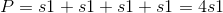## Example Questions

### Example Question #5 : Quadrilaterals

A circle with a radius 2 in is inscribed in a square. What is the perimeter of the square?

32 in

16 in

24 in

12 in

28 in

16 in

Explanation:

To inscribe means to draw inside a figure so as to touch in as many places as possible without overlapping. The circle is inside the square such that the diameter of the circle is the same as the side of the square, so the side is actually 4 in.  The perimeter of the square = 4s = 4 * 4 = 16 in.

### Example Question #2 : How To Find The Perimeter Of A Square

Square X has 3 times the area of Square Y.  If the perimeter of Square Y is 24 ft, what is the area of Square X, in sq ft?

54

108

112

72

144

108

Explanation:

Find the area of Square Y, then calculate the area of Square X.

If the perimeter of Square Y is 24, then each side is 24/4, or 6.

A = 6 * 6 = 36 sq ft, for Square Y

If Square X has 3 times the area, then 3 * 36 = 108 sq ft.

### Example Question #3 : How To Find The Perimeter Of A Square

A square has an area of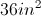.  If the side of the square is reduced by a factor of two, what is the perimeter of the new square?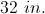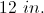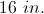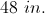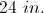Explanation:

The area of the given square is given by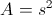so the side must be 6 in.  The side is reduced by a factor of two, so the new side is 3 in.  The perimeter of the new square is given by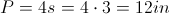.

### Example Question #4 : How To Find The Perimeter Of A Square

Find the perimeter of a square with side length 4.Explanation:

To solve, simply use the formula for the perimeter of a square.

Substitute in the side length of four into the following equation.

Thus,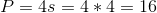### Example Question #5 : How To Find The Perimeter Of A Square

Find the perimeter of a square whose side length is 5.Explanation:

To solve, simply use the formula for the perimeter of a square. Thus,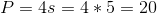### Example Question #6 : How To Find The Perimeter Of A Square

Find the perimeter of a square with side length 12.Explanation:

To solve, simply use the formula for the perimeter of the square. Thus,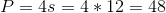If you don't remember the formula, you can simply sum the sides of a square to find the perimeter.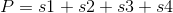However, since all the sides area the same, we get the following.